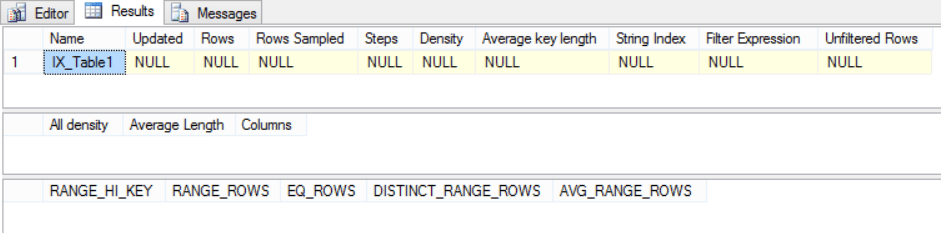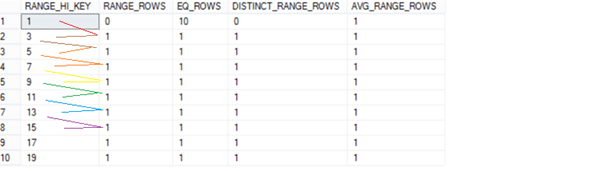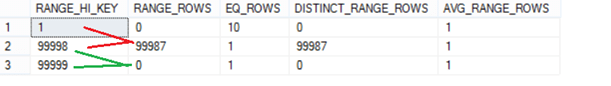# What DBCC SHOW_STATISTICS tells me

This is one of the blog post in a series of posts about optimizing SQL Server queries. A list of blog posts about query tuning can be found here – https://jbswiki.com/2021/04/15/sql-server-query-optimization-introduction/

DBCC SHOW_STATISTICS displays current query optimization statistics for a table or indexed view.  Basically it shows you the statistics, or a summary of the data, that SQL Server will use to help generate an execution plan.

=> Create below database and table.

###### Create database Test go USE Test GO CREATE TABLE [dbo].[Table1]( [Col1] [int] NULL, [Col2] [int] NULL, [Col3] [int] NULL, ) ON [PRIMARY] GO

=> You will see a clustered index created automatically. Add the below non clustered index.

###### USE Test GO CREATE NONCLUSTERED INDEX [IX_Table1] ON [dbo].[Table1] ( [Col1] ASC, [Col2] ASC, [Col3] ASC )WITH (PAD_INDEX  = OFF, STATISTICS_NORECOMPUTE  = OFF, SORT_IN_TEMPDB = OFF, IGNORE_DUP_KEY = OFF, DROP_EXISTING = OFF, ONLINE = OFF, ALLOW_ROW_LOCKS = ON, ALLOW_PAGE_LOCKS  = ON) ON [PRIMARY] GO

=> Insert some sample rows.

###### insert into Table1 values (1,2,3) go 10 set nocount on declare @i int set @i =2 while (@i<20) begin insert into Table1 values(@i,12,13) set @i=@i+1 end

=> Lets run dbcc show_statistics and see the output. We have inserted some rows, but we don’t see any output.

###### dbcc show_statistics (‘Table1′,’IX_Table1’)=> Running update statistics with fullscan and then dbcc show_statistics and we get the below output. This seems to show what we have inserted.

###### update statistics Table1 with fullscan dbcc show_statistics(‘Table1′,’IX_Table1’)Tab1 from above screenshot[\$] Name : Name of the index.

[\$] Updated : Date when the statistics for this index is updated or the time when it was last rebuilt.

[\$] Rows : Number of rows in the underlying table.

[\$] Rows Sampled : Number of rows sampled. In our case we performed an update statistics with fullscan, hence we see all the rows sampled. In most of the case, you will see less number of rows in “Rows Sampled” than the “Rows” indicating we have a sample value set.

[\$] Steps : Number of steps in the histogram. In our case it is 10, since the number of rows in tab 3 as per the above screenshot is just 10.

[\$] Density : Calculated as 1 / distinct values for all values in the first key column of the statistics object, excluding the histogram boundary values. This Density value is not used by the query optimizer and is displayed for backward compatibility with versions before SQL Server 2008.

[\$] Average Key Length : Average number of bytes per value for all of the key columns in the statistics object. In our case 3 columns are int.So, 4+4+4 = 12.

[\$] String Index : Yes indicates the statistics object contains string summary statistics to improve the cardinality estimates for query predicates that use the LIKE operator; for example, WHERE ProductName LIKE ‘%Bike’. String summary statistics are stored separately from the histogram and are created on the first key column of the statistics object when it is of type char, varchar, nchar, nvarchar, varchar(max), nvarchar(max), text, or ntext..

[\$] Unfiltered Rows : Total number of rows in the table before applying the filter expression. If Filter Expression is NULL, Unfiltered Rows is equal to Rows.

Tab2 from above screenshot.[\$] All Density : It is the value of 1/distinct rows.
select distinct col1 from Table1
=> Returns 19 rows => 1/19 = 0.05263158

select distinct col1,col2 from Table1
=> Returns 19 rows => 1/19 = 0.05263158

select distinct col1,col2,col3 from Table1
=> Returns 19 rows => 1/19 =0.05263158

[\$] Average length : Average length, in bytes, to store a list of the column values for the column prefix.

Col1 => 4
Col1, Col2 => 4+4 = 8
Col1, Col2, col3 => 4+4+4 = 12

[\$] Columns : Names of the columns in the prefix for which All density and Average length are displayed.

Tab3 from above screenshotSQL Server builds the histogram from the sorted set of column values in three steps as per https://technet.microsoft.com/en-us/library/dd535534(v=SQL.100).aspx.

=> Histogram initialization: In the first step, a sequence of values starting at the beginning of the sorted set is processed, and up to 200 values of RANGE_HI_KEY,EQ_ROWS, RANGE_ROWS, and DISTINCT_RANGE_ROWS are collected (RANGE_ROWS and DISTINCT_RANGE_ROWS are always zero during this step). The first step ends either when all input has been exhausted, or when 200 values have been found.

=> Scan with bucket merge: Each additional value from the leading column of the statistics key is processed in the second step, in sorted order; for each successive value, either the value is added to the last range, or a new range at the end is created (this is possible because the input values are sorted). If a new range is created, one pair of existing, neighboring ranges is collapsed into a single range. This pair of ranges is selected in order to minimize information loss. The number of histogram steps after the ranges are collapsed stays at 200 throughout this step. This method is based on a variation of the maxdiff histogram.

=> Histogram consolidation: In the third step, more ranges can be collapsed if a significant amount of information is not lost. Therefore, even if the column has more than 200 unique values, the histogram might have less than 200 steps.

[\$] RANGE_HI_KEY : This is the key value. This value can be anything from the below output.
select distinct col1 from Table1

[\$] RANGE_ROWS : Number of rows whose column value falls between the values as marked in below screenshot. If we take the second row, We have 1 column between RANGE_HI_KEY “1” and “3”, which is value “2”.To understand this further, insert close to 100000 rows,

###### set nocount on declare @i int set @i =29 while (@i<100000) begin insert into Table1 values(@i,12,13) set @i=@i+1 end

Now check the output of TAB3 again,It is pretty evident, that between RANGE_HI_KEY “1” and “99998” we have 99987 rows.

[\$] EQ_ROWS : Total number of rows equal to corresponding RANGE_HI_KEY.
From the above screenshot,

Row 1 => RANGE_HI_KEY is 1 => select COUNT(*) from table1 where col1=1 => 10, which is equal to EQ_ROWS.

Row 2 => RANGE_HI_KEY is 99998 => select COUNT(*) from table1 where col1=99998 => 1, which is equal to EQ_ROWS.

Row 3 => RANGE_HI_KEY is 99999 => select COUNT(*) from table1 where col1=99999 => 1, which is equal to EQ_ROWS.

[\$] DISTINCT_RANGE_ROWS : Number of distinct rows between each of the values in RANGE_HI_KEY. From the above screenshot,

Row 1 => RANGE_HI_KEY is 1 => This is the first row and it will always be 0.

Row 2 => RANGE_HI_KEY is 99998 => select distinct count(col1) from table1 where col1>1 and col1<99998 => 99987, which is equal to  DISTINCT_RANGE_ROWS.

Row 3 => RANGE_HI_KEY is 99999 => select distinct count(col1) from table1 where col1>99998 and col1<99999 => 0, which is equal to DISTINCT_RANGE_ROWS.

[\$] AVG_RANGE_ROWS : Average number of rows with duplicate column values within a histogram step, excluding the upper bound (RANGE_ROWS / DISTINCT_RANGE_ROWS for DISTINCT_RANGE_ROWS > 0).

Row 1 => RANGE_HI_KEY is 1 => DISTINCT_RANGE_ROWS = 0, Hence AVG_RANGE_ROWS is 1.

Row 2 => RANGE_HI_KEY is 99998 => DISTINCT_RANGE_ROWS > 0, (RANGE_ROWS / DISTINCT_RANGE_ROWS) =>(99987 / 99987) => 1, which is same as AVG_RANGE_ROWS.

Row 3 => RANGE_HI_KEY is 99999 => DISTINCT_RANGE_ROWS = 0, Hence AVG_RANGE_ROWS is 1.

Thank You,
Vivek Janakiraman

Disclaimer:
The views expressed on this blog are mine alone and do not reflect the views of my company or anyone else. All postings on this blog are provided “AS IS” with no warranties, and confers no rights.